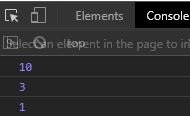Related Articles
Underscore.js _.random() Function
• Last Updated : 14 Jul, 2020

Underscore.js is a library in javascript that makes operations on arrays, string, objects much easier and handy.
The _.random() function is used to return a random integer which is in the range provided to the function.

Note: It is very necessary to link the underscore CDN before going and using underscore functions in the browser. When linking the underscore.js CDN The “_” is attached to the browser as a global variable.

Syntax:

`_.random(min, max);`

Parameters: It takes the following parameters.

• min value: It is the minimum random value to be returned by the function.
• max value: It is the maximum random value that can be returned by the function.

Return Value: It returns a random integer between the min and max. If only one value is passed then it will return random value between 0 and passed value.

Example 1:

 ```<``html``>`` ` `<``head``>``    ``<``script` `src``=``"https://cdnjs.cloudflare.com/ajax/libs/underscore.js/1.9.1/underscore-min.js"``>``    `````` ` `<``body``>``    ``<``script``>``        ``let min = 5;``        ``let max = 10;`` ` `        ``// Printing 5 random values``        ``// in range 5 and 10``        ``for (let i = 0; i < ``5``; i++) {``            ``console.log(_.random(min, max));``        ``}``    `````` ` ``

Output:Example 2: If no parameter is given to the random function.

 ```<``html``>`` ` `<``head``>``    ``<``script` `src``=``"https://cdnjs.cloudflare.com/ajax/libs/underscore.js/1.9.1/underscore-min.js"``>``    `````` ` `<``body``>``    ``<``script``>`` ` `        ``// Without passing any ``        ``// value to function``        ``console.log(_.random())``    `````` ` ``

Output:Example 3: When only one parameter is given then random value is in range 0 to n, where n is the parameter given.

 ```<``html``>`` ` `<``head``>``    ``<``script` `src``=``"https://cdnjs.cloudflare.com/ajax/libs/underscore.js/1.9.1/underscore-min.js"``>``    `````` ` `<``body``>``    ``<``script``>``        ``console.log(_.random(10))``        ``console.log(_.random(5))``        ``console.log(_.random(8))``    `````` ` ``

Output:My Personal Notes arrow_drop_up